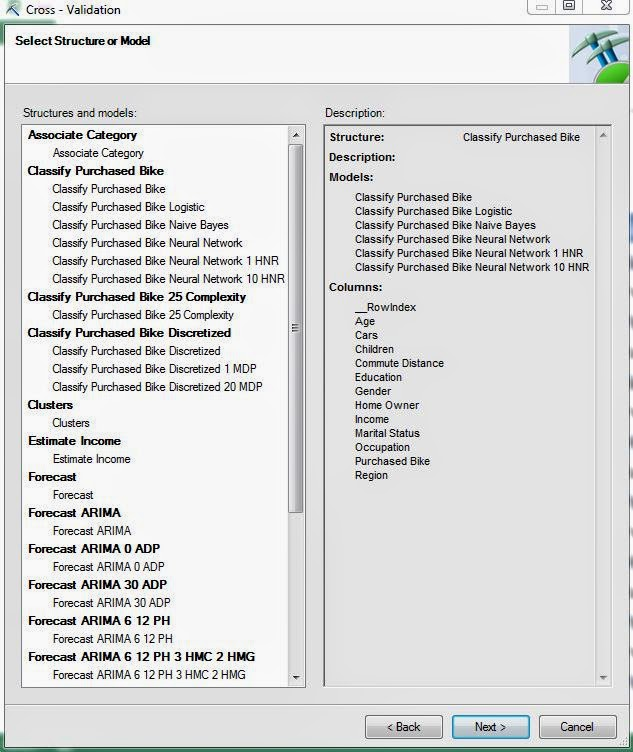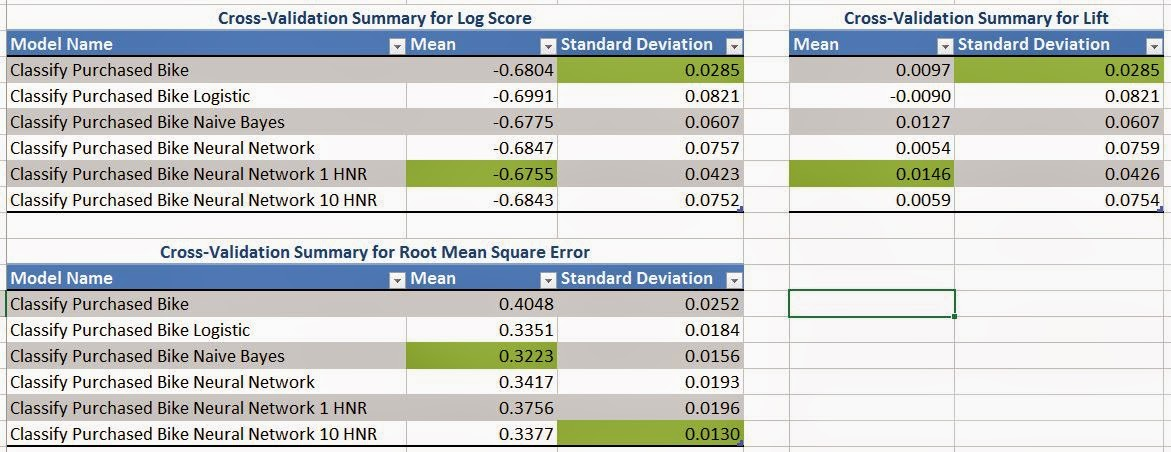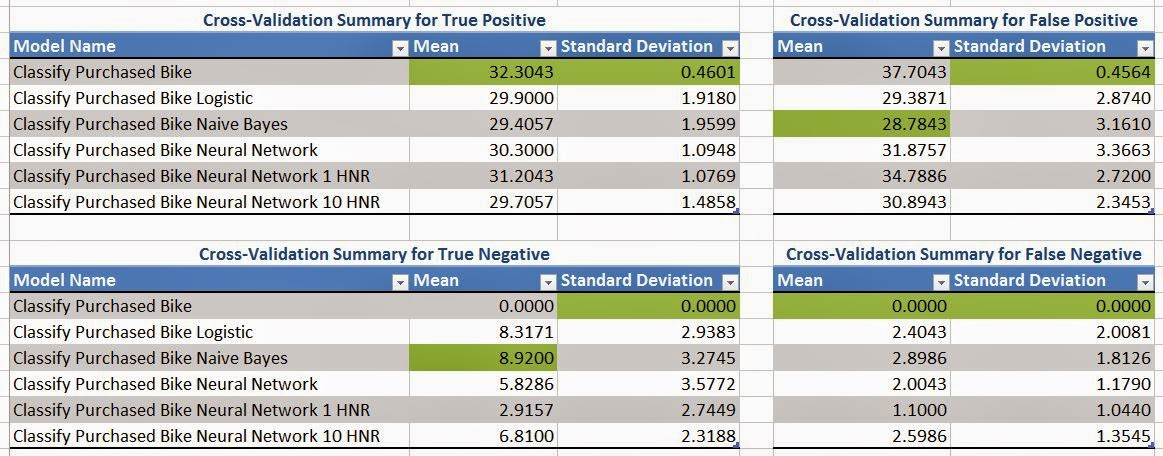## Monday, November 24, 2014

### Data Mining in Excel Part 30: Cross-Validation

Today, we're going to talk about the final tool in the "Accuracy and Validation" segment of the Data Mining Ribbon, Cross-Validation.Cross-Validation
Imagine you have a data set with 10,000 rows.  Then, you randomly assign 1,000 rows to each of 10 buckets.  Next, you create a model using 9 of the buckets (9000 rows) as training data and 1 bucket (1000 rows) as testing data.  Then, you repeat this process for the using each of the remaining 9 buckets as testing data.  After this is done, you have 10 models using parts of the same data set, which means you have 10 different predictions for each row.  Then, you can compare the predictions and see how much they deviate from each other.  If they are all very close to each other, then the model is "good".  If some of them are very far apart, then the model may not be appropriate for the data you have.  You can also compute a single prediction by combining all or part of your 10 predictions into a single value.  This process is called Cross-Validation.  For more information, read this.  Let's see it in action.Select Structure
First, we need to select the structure or model we would like to use.  Unlike the previous methods, this technique can technically use Association Rules Models as well.  However, the structure must have a variable with the "Predict" flag, which doesn't come from the Association Rules Model.  Therefore, we're not sure how you could use this technique on an Association Rules Model.  Let's move on.Select Parameters
Next, we need to select our parameters to be used.  Fold Count refers to the number of partitions we create using the data.  In our earlier example, we used 10.  You also need to define how many rows you would like to be used for consideration.  The larger the number of rows, the longer the algorithm will take.  Then you need to define with value you are looking, Purchased Bike = Yes in our case.  Finally, you need to decide what probability defines a "True".  These algorithms don't actually assign a True/False value to the field, they assign a probability.  The higher the probability, the more likely the customer will buy a bike.  For now, let's just set this value at .5, like the flip of a coin.  Finally, let's check out the results.Prediction Summary (.5 TT)
We rearranged the tables to make it easier to read.  Here, we have tables telling us the average number of true positives, false positives, true negatives, and false negatives across the 10 folds, as well as the associated standard deviations.  We want to have high numbers for True scores and low number for False scores.  We also want standard deviations to be small because this implies that the models are robust, i.e. aren't heavily influenced by differences in the data.  Looking at this carefully, we see that the Naive Bayes Model performs well on all fronts and has a pretty low standard deviation.  This, combined with the fact that this model also performed well on the other previous Model Validation tests, leads us to believe that the Naive Bayes Model is a good fit for this type of analysis.  However, this is just our opinion and is definitely up to interpretation.  Let's check out the next set of metrics further down the sheet.Metric Summary (.5 TT)
Here, we have 3 metrics displayed, Log Score, Lift, and Root Mean Squared Error.  We won't go into detail about what these metrics mean.  If you want to know you can read Log Score, Lift, and Root Mean Squared Error.  Basically, we want Log Score and Lift to be high, while keeping Root MSE low.  Of course, we also want the standard deviations to be small.  Personally, we don't feel like these metrics are as important as the prediction values we saw previously.  So, we'll just use them to confirm that the Naive Bayes Model is reasonable, and they do.  Before we finish, let's check the Prediction Summary for different values of Target Threshold to ensure stability of our Naive Bayes Model.Prediction Summary (.2 TT)Prediction Summary (.8 TT)
By looking at these charts, we see that the Naive Bayes Model seems reasonable in all cases.  This leads us to conclude that the Naive Bayes Model is the model we will use to predict which customers will buy our bikes.  Stay tuned next week where we'll be talking about our final topic, Querying your Models.  Thanks for reading.  We hope you found this informative.